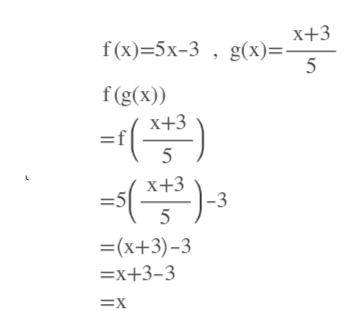Determine algebraically whether f and g are inverse functions. f(x) = 5x - 3          g(x)= x+3/5

Question
Determine algebraically whether f and g are inverse functions.

f(x) = 5x - 3          g(x)= x+3/5

Step 1

To show f and g are inverse functions, we have to show f(g(x))=x and g(f(x))=x.

Step 2

Here we have shown t...help_outlineImage Transcriptioncloseх+3 f (x)-5х-3 , g(х)%. f(g(x)) х+3 5 х+3 -3 -5 5 =(x+3)-3 —х+3-3 3Dх fullscreen

Want to see the full answer?

See Solution

Want to see this answer and more?

Our solutions are written by experts, many with advanced degrees, and available 24/7

See Solution
Tagged in

Algebra